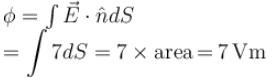Courses

# Gauss Law And Field Due To Charge Distribution MCQ

## 10 Questions MCQ Test Topic wise Tests for IIT JAM Physics | Gauss Law And Field Due To Charge Distribution MCQ

Description
This mock test of Gauss Law And Field Due To Charge Distribution MCQ for IIT JAM helps you for every IIT JAM entrance exam. This contains 10 Multiple Choice Questions for IIT JAM Gauss Law And Field Due To Charge Distribution MCQ (mcq) to study with solutions a complete question bank. The solved questions answers in this Gauss Law And Field Due To Charge Distribution MCQ quiz give you a good mix of easy questions and tough questions. IIT JAM students definitely take this Gauss Law And Field Due To Charge Distribution MCQ exercise for a better result in the exam. You can find other Gauss Law And Field Due To Charge Distribution MCQ extra questions, long questions & short questions for IIT JAM on EduRev as well by searching above.
QUESTION: 1

### If the flux of the electric field through a closed surface is zero

Solution:

Flux across a closed surface,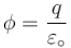Since,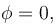so, charge enclosed q = 0

The correct answer is: the net charge inside the surface must be zero

QUESTION: 2

### Electric charge is uniformly distributed along a long straight wire of radius 1mm. The charge per cm length of the wire is Q  coulomb. Another cylindrical surface of radius 50cm and length 1m symmetrically encloses the wire as shown in figure. The total electric flux passing through the cylinder surface is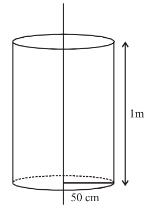Solution: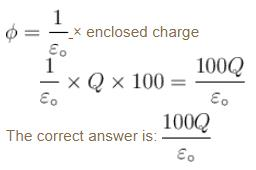QUESTION: 3

### A sphere of radius R has a uniform of volume charge density. The electric potential at a point r, r < R  is

Solution: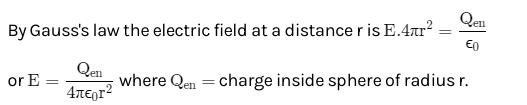As the potential V=−∫Edr so the electric potential at r due to charge inside a sphere of radius r only.

QUESTION: 4

Charge on the outer sphere is q and the inner sphere is grounded. Then the charge q' on the inner sphere r2 > r1.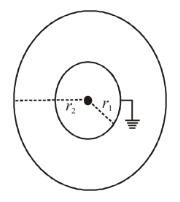Solution:

Potential at the surface of inner sphere = 0 (grounded)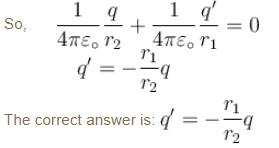QUESTION: 5

If the electric flux entering and leaving an enclosed surface respectively is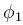and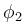the electric charge inside the surface will be

Solution: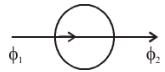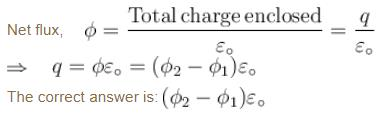QUESTION: 6

The SI unit of electric flux is

Solution:

Electric flux  = E.A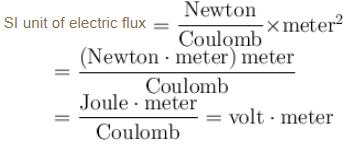The correct answer is: volt × metre

QUESTION: 7

A point charge is kept at the mid point between two large parallel grounded conducting plates. Assuming no gravity. The charge is displaced a little towards the right plate. The charge will now

Solution:

When the charge is placed at the mid point, opposite charge will induced on both the plates equally. But when q is displaced toward the right, more charge of the opposite sign will get induced on the right plate than on the left plate. Hence the point charge will feel a net attraction towards the right plate.

The correct answer is: Move towards the right plate

QUESTION: 8

The figure shows electric field E at a distance r in any direction from the origin O. The electric field is due to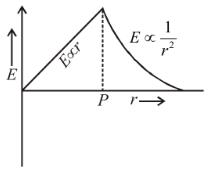Solution:

From Gauss Law of electrostatics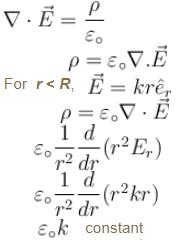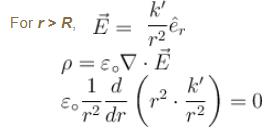The correct answer is: a uniform charged non-conducting sphere of radius OP  with centre at O.

QUESTION: 9

A charge Q is enclosed by a Gaussian spherical surface of radius R. If the radius is doubled, then the outward electric flux will

Solution: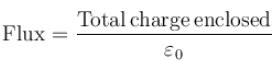Since, flux across a closed surface depends only on the charge enclosed. So, it will remain same

The correct answer is: remain the same

QUESTION: 10

Given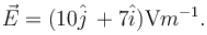The electric flux through 1m2 area in YZ plane is

Solution:

For yz plane,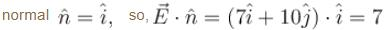So, flux across yz plane is given by